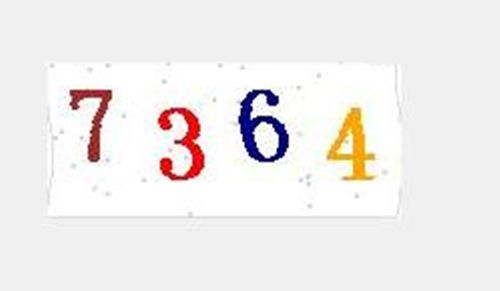# 在Python中使用tesseract识别验证码

## 前言

• 第一种，最简单也是最费时的，手动输入验证码；

• 第二种，使用一些公司的API接口对验证码进行判别和输入；

• 第三种，使用tessract对验证码进行识别；

## Tesseract简介

tesseract是谷歌开源的一个ORC组件，并支持语言的训练，支持中文的识别（需要下载语言包）

## Python中使用Tesseract

### 1、pip安装pytesseract及其他依赖库

``````pip pytesseract
``````

### 3、修改tesseract.py文件

``````# tesseract_cmd = 'tesseract'
tesseract_cmd = "C:/Program Files (x86)/Tesseract-OCR/tesseract.exe" # tesseract的安装目录
``````

``````# f = open(output_file_name)
f = open(output_file_name,encoding='utf-8')
````````````#coding:utf-8
'''
验证码识别
'''
from PIL import Image,ImageFilter,ImageEnhance
import pytesseract

# 二值化
threshold = 140
table = []
for i in range(256):
if i < threshold:
table.append(0)
else:
table.append(1)

# 识别验证码
def get_vcode():
# 打开原始图像
image = Image.open("getimgbysig.jpg")
# image = Image.open("e:/a.jpg")

# 将图像转为灰度，并另存为
bimage = image.convert('L')
bimage.save('g'+"getimgbysig.jpg")

# 进行二值化处理，并另存为
out = bimage.point(table,'1')
out.save('b'+"getimgbysig.jpg")

icode = pytesseract.image_to_string(image)
bcode = pytesseract.image_to_string(bimage)
vcode = pytesseract.image_to_string(out)
print(icode,bcode,vcode)

if __name__ == '__main__':
get_vcode()
``````

## 猜你也喜欢

1.匿名说道：

2.匿名说道：